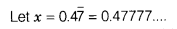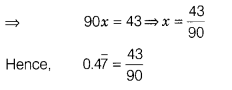# Express 0.47 in the form p\q, where p and q are integers and q= 0

Express 0.47 in the form p\q, where p and q are integers and q= 0.eq(i)
Multiplying by 10 both sides,we get
10x=4.7777…eq(ii)
Multiplying again by 10 both sides,we get
100x=47.7777…eq(iii)
On subtraction eq.(ii) from eq.(iii),we get
(100x-10x)=(47.7777…)-(4.7777)